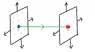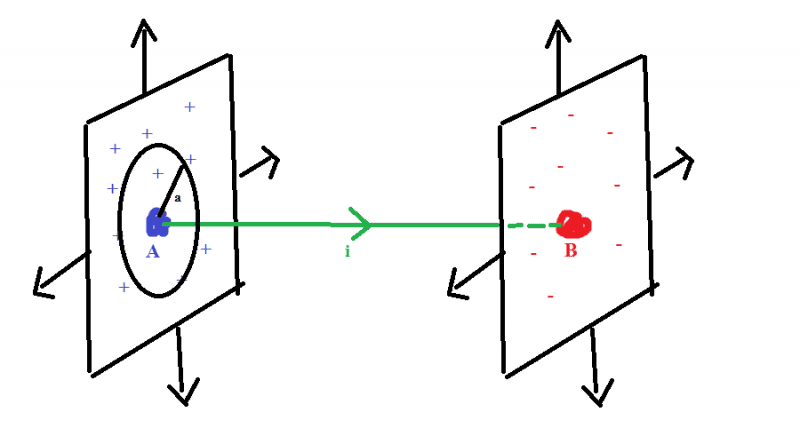# Problems about Ampere's Law and Biot-Savart's Law

arpon
Suppose there is a potential difference between points A and B which are connected by a straight wire. The current in AB is $i$.
We want to calculate the magnetic field at point C which is at distance $r$ from the middle point P of the wire and CP is perpendicular to AB.
At first, we use Ampere's law. We consider a circular loop (of radius $r$) around the wire. Because of symmetry, magnetic field is always same in this loop. As the current through this loop is $i$,we get,
$\oint \vec B \cdot d \vec s = B(2\pi r) = \mu _0 i$
So, $B = \frac {\mu _0 i}{2\pi r}$
But, now we use Biot-Savart's law.
$dB = \frac {\mu _0 i}{4\pi } \frac {i dl sin\theta }{r^2 + l^2}$
So, $B = \frac {\mu _0 i}{4\pi r} \frac {L}{\sqrt {r^2 + (\frac{L}{2})^2}}$, where $L =$the length of AB.

Suppose there is a potential difference between points A and B which are connected by a straight wire. The current in AB is $i$.
We want to calculate the magnetic field at point C which is at distance $r$ from the middle point P of the wire and CP is perpendicular to AB.
At first, we use Ampere's law. We consider a circular loop (of radius $r$) around the wire. Because of symmetry, magnetic field is always same in this loop. As the current through this loop is $i$,we get,
$\oint \vec B \cdot d \vec s = B(2\pi r) = \mu _0 i$
So, $B = \frac {\mu _0 i}{2\pi r}$
But, now we use Biot-Savart's law.
$dB = \frac {\mu _0 i}{4\pi } \frac {i dl sin\theta }{r^2 + l^2}$
So, $B = \frac {\mu _0 i}{4\pi r} \frac {L}{\sqrt {r^2 + (\frac{L}{2})^2}}$, where $L =$the length of AB.
The expression is for infinitely long wire. You will get same expression from both laws.

Mentor
Suppose there is a potential difference between points A and B which are connected by a straight wire. The current in AB is ii.

If the wire has finite length (begins at A and ends at B), then while the current flows, there is an increasing charge at one end and a decreasing charge at the other end, because of charge conservation. These time-varying charges produce time-varying electric fields which are associated with magnetic fields according to Maxwell's extension of Ampere's Law (the "displacement current"): $$\oint {\vec B \cdot d \vec l} = \mu_0 \iint {\vec J \cdot d \vec a} + \mu_0 \epsilon_0 \frac{d}{dt} \iint {\vec E \cdot d \vec a}$$ The second term on the right side contains the "displacement current". $$\oint {\vec B \cdot d \vec l} = \mu_0 (i + i_\textrm{disp})$$ where $$i_\textrm{disp} = \epsilon_0 \frac{d}{dt} \iint {\vec E \cdot d \vec a}$$

Last edited:
•Without making things complicated, for wire having finite length and if you want to find out the magnetic field at a point at distance r from wire,
Take a small length dl in wire.
$$dB = \frac{\mu_0}{4\pi}\frac{idlsin\theta}{r^2}$$
Let's say the angle between lines connecting the centre of wire and point be ##\theta_1## and ##\theta_2##
##l=Rcot(\pi-\theta)##
##dl=Rcosec^2\theta d\theta##
Now,
$$dB=\frac{\mu_0}{4 \pi}\frac{isin\theta .Rcosec^2\theta d\theta}{R^2cosec^2\theta}$$
After integrating from ##\theta_1## to ##\pi-\theta_2## you will get
$$B=\frac{\mu_0 I}{4\pi R}(cos \theta_1 + cos\theta_2)$$

arpon
If the wire has finite length (begins at A and ends at B), then while the current flows, there is an increasing charge at one end and a decreasing charge at the other end, because of charge conservation. These time-varying charges produce time-varying electric fields which are associated with magnetic fields according to Maxwell's extension of Ampere's Law (the "displacement current"):
[I have attached a photo with it to explain my problem.]
Suppose, the points A and B are situated on two infiinite plates, one is positively charged and the other negative.
As the plates are infinite, the electric field, $E = \frac{\sigma }{\epsilon _0}$ ; ($\sigma$is the density of charge)
So, if charge is added in the plates or removed, density of charge won't change , because the plates are infinite.
So, there is no change of $E$ , so the displacement current is zero.

#### Attachments

•Untitled.png
8.2 KB · Views: 613
Last edited:
•Mentor
There are radial currents in both plates, because charge arriving at point B has to flow outwards to the rest of that plate, and charge leaving point A has to flow inwards from the rest of that plate.

•arpon
arpon
There are radial currents in both plates, because charge arriving at point B has to flow outwards to the rest of that plate, and charge leaving point A has to flow inwards from the rest of that plate.
I got your point. But four questions arises in my mind:
1. How to calculate the radial current?
To solve this, I think I need to apply Kirchhoff's law. The current that flows through the wire AB, should be equal to the radial current. If the resistence of the wire AB is $R$ and the resistence in the plates is negligeble, the current $i$ should be $\frac{V}{R}$ , where, $V =$the potential difference between A and B (or the plates). Am I right?

2. Then I thought about the drift velocity of charge (let it be $v_d$) in the plate. Suppose the positive charge moves, for simplicity. [I attached a photo in this case]I assumed a circle (let its radius be $a$) which has its center on A in the plate; Because of symmetry, the drift velocity of the charges on the circle is equal (in quantity) and the direction will be towards the center. So, we may write,
$i = \sigma v_d * 2\pi a$
(I am not clear why it is so. But as the dimension on two sides are equal, I assume it is so. Please also explain this matter)
Then,
$v_d = \frac{i}{\sigma * 2\pi a}$
But at the center A, the drift velocity becomes undefined.

3. Now, think about the problem I mentioned earlier (about calculating the magnetic field). If we consider a loop around the wire, the radial current does not pass through the surface enclosed by the loop. So, would it be, $B$ is equal to $\frac{\mu _0 i}{2\pi r}$ just same as the case of infinite wire?

4. In Biot-Savart's law, there is nothing about the influence on magnetic field because of the change in electric field (displacement current). So, when there is a change in electric field, can't we apply it?

Please, explain these points in details.

Mentor
Hi, I'm sorry for the delay. This thread dropped off my radar, so to speak, because of holiday activities.I got your point. But four questions arises in my mind:
1. How to calculate the radial current?
To solve this, I think I need to apply Kirchhoff's law. The current that flows through the wire AB, should be equal to the radial current. If the resistence of the wire AB is $R$ and the resistence in the plates is negligeble, the current $i$ should be $\frac{V}{R}$ , where, $V =$the potential difference between A and B (or the plates). Am I right?

Correct, under your assumption that the resistance in the plates is negligible compared to the resistance in the wire.

2. Then I thought about the drift velocity of charge (let it be $v_d$) in the plate. [...] But at the center A, the drift velocity becomes undefined.

Real wires have a finite radius, so in practice you never reach the center.

3. Now, think about the problem I mentioned earlier (about calculating the magnetic field). If we consider a loop around the wire, the radial current does not pass through the surface enclosed by the loop.

An infinite number of surfaces, of different shapes, have the loop as their boundary. Ampere's Law applies to all of them. If the surface is a flat disk, then only the current in the wire passes through the surface. Now imagine a surface that is a cylinder that is open at one end (the end where the loop is), and extends through one of the plates. The current in the wire does not pass through this surface, but the radial current in the plate does. However, as you noted earlier, the total radial current must equal the current in the wire. Therefore, the following statement is true:

So, would it be, $B$ is equal to $\frac{\mu _0 i}{2\pi r}$ just same as the case of infinite wire?

4. In Biot-Savart's law, there is nothing about the influence on magnetic field because of the change in electric field (displacement current). So, when there is a change in electric field, can't we apply it?

Correct, Biot-Savart applies only when the effects of a changing electric field are negligible.

•arpon